Courses

# Design of Bearings - MCQ Test 2

## 30 Questions MCQ Test GATE Mechanical (ME) 2022 Mock Test Series | Design of Bearings - MCQ Test 2

Description
This mock test of Design of Bearings - MCQ Test 2 for Mechanical Engineering helps you for every Mechanical Engineering entrance exam. This contains 30 Multiple Choice Questions for Mechanical Engineering Design of Bearings - MCQ Test 2 (mcq) to study with solutions a complete question bank. The solved questions answers in this Design of Bearings - MCQ Test 2 quiz give you a good mix of easy questions and tough questions. Mechanical Engineering students definitely take this Design of Bearings - MCQ Test 2 exercise for a better result in the exam. You can find other Design of Bearings - MCQ Test 2 extra questions, long questions & short questions for Mechanical Engineering on EduRev as well by searching above.
QUESTION: 1

### The dynamic load capacity of 6306 bearing is 22 kN. The maximum radial load it can sustain to operate at 600 rev/min, for 2000 hours is

Solution: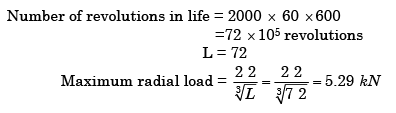QUESTION: 2

### Which one of the following is a criterion in the design of hydrodynamic journal bearings?

Solution:

Sommerfeld Number, also Known as bearing Characteristic Number,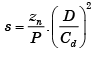QUESTION: 3

### A natural feed journal bearing of diameter 50 mm and length 50 mm operating at 20 revolution/second carries a load of 2.0 KN. The lubricant used has a viscosity of 20 mPas. The radial clearance is 50 μm. The Sommerfeld number for the bearing is

Solution: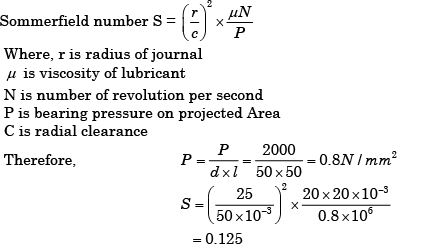QUESTION: 4

Starting friction is low in

Solution:
QUESTION: 5

Average values of effective coefficients of friction for bearings are described below: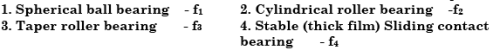Which one of the following sequences is correct?

Solution: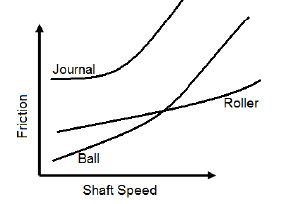QUESTION: 6

Which one of the following statements is correct?

Antifriction bearings are

Solution:
QUESTION: 7

The rolling element bearings are

Solution:
QUESTION: 8

Ball bearings are provided with a cage

Solution:
QUESTION: 9

Which one of the following statements is NOT true of rolling contact bearing?

Solution:
QUESTION: 10

Consider the following statements about anti-friction bearings:

1. They have low starting and low running friction at moderate speeds.

2. They have high resistance to shock loading.

3. They can carry both radial and thrust loads.

4. Their initial cost is high.

5. They can accommodate some amount of misalignments of shaft.

Of these statements

Solution:

Self aligning bearing can accommodate some amount of misalignments of shaft.

QUESTION: 11

If the load on a ball bearing is halved, its life:

Solution: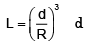is dynamic load carrying capacity. R is actual load applied if R halved L will increased by 23 = 8 times

QUESTION: 12

Which bearing is preferred for oscillating conditions?

Solution:
QUESTION: 13

Match List-I with List-II and select the correct answer using the codes given below the lists: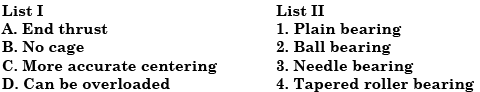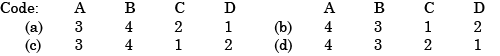Solution:
QUESTION: 14

In a collar thrust bearing, the number of collars has been doubled while maintaining coefficient of friction and axial thrust same. It will result in

Solution:
QUESTION: 15

In sliding contact bearings, a positive pressure can be built up and a load supported by a fluid only by the use of a:

Solution:
QUESTION: 16

Increase in values of which of the following results in an increase of the coefficient of friction in a hydrodynamic bearing?

1. Viscosity of the oil.

2. Clearance between shaft and bearing.

3. Shaft speed.

Select the correct answer using the code given below:

Solution:

2 is false  Petroff’s law says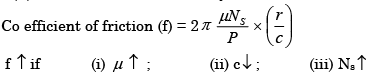QUESTION: 17

Consider tile following statements:

Radius of friction circle for a journal bearing depends upon

1. Coefficient of friction

2. Radius of the journal

3. Angular speed of rotation of the shaft

Which of the statements given above are correct?

Solution:

radius of friction circle = f ×r

QUESTION: 18

In a journal bearings, the radius of the friction circle increases with the increase in

Solution:
QUESTION: 19

The bearing characteristic number in a hydrodynamic bearing depends on

Solution:
QUESTION: 20

Assertion ( A): In equilibrium position, the journal inside a journal bearing remains floating on the oil film.

Reason (R): In a journal bearing, the load on the bearing is perpendicular to the axis of the journal.

Solution:

Both A and R are true but R is not correct explanation for A.

QUESTION: 21

A sliding contact bearing is operating under stable condition. The pressure developed in oil film is p when the journal rotates at N r.p.m. The dynamic viscosity of lubricant is μ and effective coefficient of friction between bearing and journal of diameter D is f. Which one of the following statements is correct for the bearing?

Solution: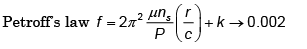QUESTION: 22

Consider the following pairs of types of bearings and applications:

1. Partial Journal bearing………………. Rail wagon axles

2. Full journal bearing ………………….Diesel engine crank-shaft

3. Radial bearing ……………………….Combined radial and axial loads

Which of these pairs is/are correctly matched?

Solution:
QUESTION: 23

Assertion (A): In anti-friction bearings, the frictional resistance is very low as the shaft held by it remains in floating condition by the hydrodynamic pressure developed by the lubricant.

Reason (R): In hydrodynamic journal bearings, hydrodynamic pressure is developed because of flow of lubricant in a converging -diverging channel

Solution:
QUESTION: 24

In an oil-lubricated journal bearing, coefficient of friction between the journal and the bearing.

Solution:
QUESTION: 25

Which one of the following pair is correctly matched?

Solution:
QUESTION: 26

Which one of the following types of bearings is employed in shafts of gearboxes of automobiles?

Solution:
QUESTION: 27

Assertion (A): In hydrodynamic journal bearings, the rotating journal is held in floating condition by the hydrodynamic pressure developed in the lubricant.

Reason (R): Lubricant flows in a converging-diverging channel.

Solution:

Both A and R are true and R provides correct explanation for A

QUESTION: 28

Deep groove ball bearings are used for

Solution:

Deep groove ball bearings are primarily designed to support radial loads at high speeds. However, this type of construction permits the bearing also to support relatively high thrust loads in either direction.

QUESTION: 29

In a journal bearing P = average bearing pressure, Z = absolute viscosity of the lubricant, N = rotational speed of the journal. The bearing characteristic number is given by

Solution:
QUESTION: 30

Match List-I (Applications) with List-II (Choice of Bearings) and select the correct answer using the codes given below the lists: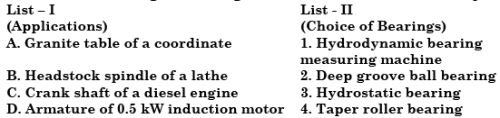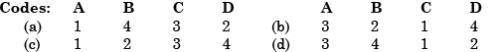Solution: